﻿ 16074306-唐书娟-第四次作业 - 计算数学达人 - 专，学者，数值代数，微分方程数值解

### 16074306-唐书娟-第四次作业

1、假设一般罪犯作案范围不会很大，基本控制在一两个城市之间。

2、假设连环案作案是单人行动，不考虑团伙作案。

3、假设罪犯作案地点基本选择在自己的居住地附近。

4、假设罪犯居住地不会改变且只有一个居住地。

5、假设罪犯活动不受地势和交通影响。

1、“圆周假设”理论：假设一个犯罪嫌疑人连续作案，及作案地点分布广泛，若找出两个最远的犯罪位置，将二者连接起来并以此直线为直径，画出一个包括所有犯罪地点的圆周，多数情况下，犯罪嫌疑人就住在圆周里，而且可能就住在靠近圆周中心的地区。犯罪圆周假设的根基是基于犯罪人的行为、生理、心里以及期活动期间地理背景的根基。“犯罪圆周假设”：犯罪分子首次作案，由于缺乏经验，加上心理恐惧的作用，极有可能选择离自己居住地比较近比较熟悉的地方作案。但随着作案次数的增多，作案经验的丰富，第二次作案会比第一次作案远，第三次作案比第二次远。总会潜意识逃避自己藏匿地点。但是有意识逃避终归要有一个限度，到了其不愿去或过于偏远的地方，出于自身考虑犯罪分子只能改变方向，以其所住为中心，向周边扩展。这样作案轨迹就由直线变为扇形最后变为圆周型如图1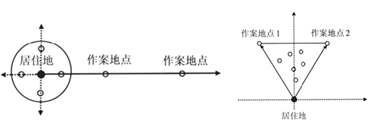1 犯罪圆周假设

2、圆周假设理论：

3、中心图解法：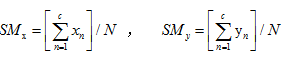SMx是空间平均值x轴坐标，SMy是空间平均值y轴坐标，N是指案发地点总数，xn,yn是指第n个犯罪地点的坐标。

SMx,SMy）即为所有犯罪地点通过中心图解法算出的中心，也就是预测的可能居住地。

4、改进最匹配圆模型：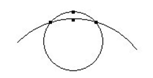2

S内任意一点A(x,y),A到所有案发地点的距离之和L。遍历S中所有点找出使得L最小的点A(x,y)。即：罪犯极可能居住在A点附近。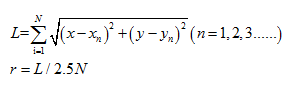N  是案发地点数目；

r    是最匹配圆的半径，也是下面Rossmo公式的缓冲半径。

5Rossmo公式预测犯罪人居住地模型

Rossmo公式构造的模型是一种被普遍用来预测罪犯居住地的方法。首先将需要处理的地区划分为i*j个小区域，运用Rossmo公式求得罪犯居住地在第ij个小区域的可能性，以此来确定罪犯的最可能的居住地范围。

Rossmo 公式：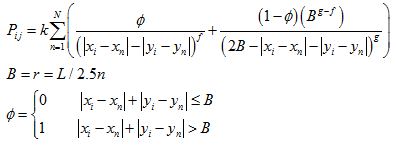Pij是居住地在区域的可能性，N是案件发生总数，xi,yi是区域的坐标，xn,yn是第个犯罪地点的坐标，B是缓冲区域的半径，即最匹配圆半径，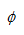是一个权重系数，k是一个经验决定的常数，fg是一个经验决定的指数，用来调节距离影响，r是最匹配圆半径。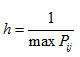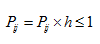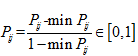6、预测下次作案时间和地点的GM11）预测模型

1）灰色预测理论：灰色预测理论是整个灰色系统理论的重要组成部分，建立灰色动态模型是灰色预测理论的核心。灰色预测模型其实质是将一组可能杂乱无章的原始序列，通过累加生成或其他运算生成呈现一定规律的序列。

2）模型的建立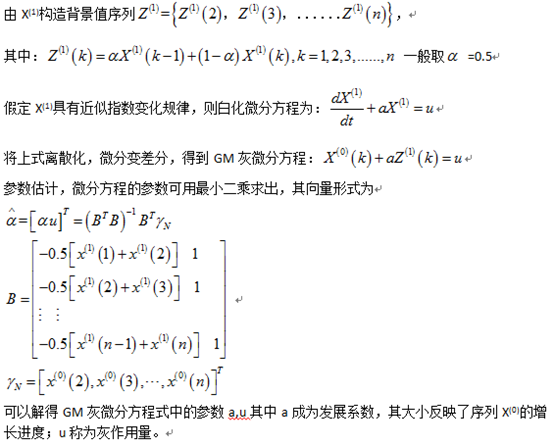X(1)的预测模型为：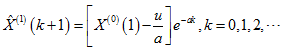X(0)的预测模型为：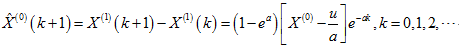3）模型的修正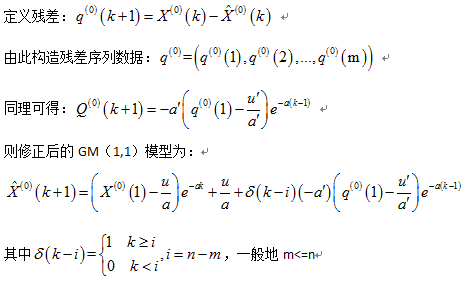1、将案发地点转化为坐标图如图3matlab代码如下：

A=[    0.8832    0.4020

0.7994    0.5721

0.7423    0.3525

0.8083    0.6609

1.1192    0.3386

1.1243    0.3069

1.1675    0.3462

1.1713    0.3360

0.9009    0.4236

1.1294    0.3259

0.8933    0.3767

0.1179    0.0353

1.1142    0.3195

0.8806    0.4084

0.7639    0.1711

0.1661    0.0556

1.0570    0.4109

0.7144    0.3081

0.8857    0.3881

1.0875    0.3538

1.1002    0.3272

0.7613    0.2244

1.0177    0.3525

];

plot(A(:,1),A(:,2),'x','LineWidth',2)%所有案发点坐标图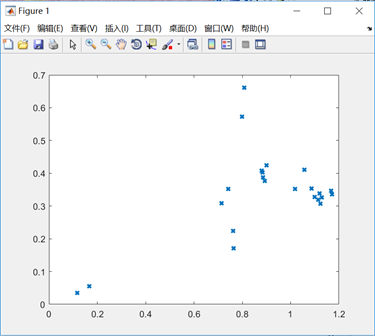3居住地点坐标图

2、中心图解法求解：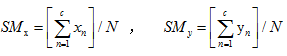Matlab代码：

A=[    0.8832    0.4020

0.7994    0.5721

0.7423    0.3525

0.8083    0.6609

1.1192    0.3386

1.1243    0.3069

1.1675    0.3462

1.1713    0.3360

0.9009    0.4236

1.1294    0.3259

0.8933    0.3767

0.1179    0.0353

1.1142    0.3195

0.8806    0.4084

0.7639    0.1711

0.1661    0.0556

1.0570    0.4109

0.7144    0.3081

0.8857    0.3881

1.0875    0.3538

1.1002    0.3272

0.7613    0.2244

1.0177    0.3525

];

a=sum(A(:,1))/23;

b=sum(A(:,2))/23;

plot(A(:,1),A(:,2),'x','LineWidth',2)%所有案发点坐标图

hold on

plot(a,b,'-ro',0.88,0.42,'+')%画出模型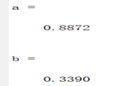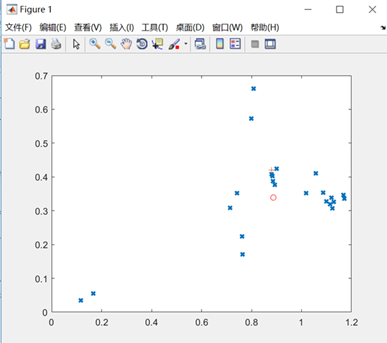4

3、最匹配圆求解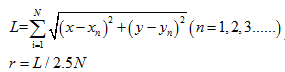matlab计算得到使最小的居住点坐标A0.89300.3460），此时最匹配圆半径为r=0.0934。得到的结果与实际居住地比较接近。作图可得以求得的居住地A为圆心，半径r=0.0934的居住地范围。

Matlab代码：

A=[    0.8832    0.4020

0.7994    0.5721

0.7423    0.3525

0.8083    0.6609

1.1192    0.3386

1.1243    0.3069

1.1675    0.3462

1.1713    0.3360

0.9009    0.4236

1.1294    0.3259

0.8933    0.3767

0.1179    0.0353

1.1142    0.3195

0.8806    0.4084

0.7639    0.1711

0.1661    0.0556

1.0570    0.4109

0.7144    0.3081

0.8857    0.3881

1.0875    0.3538

1.1002    0.3272

0.7613    0.2244

1.0177    0.3525

];

x=zeros(1,2);d=0;x1=A(:,1);y1=A(:,2);d1=zeros(1,23);dsum=inf;

for a=0:0.001:1.2

for b=0.:0.001:0.7

for i=1:23

d1(i)=abs(a-x1(i))+abs(b-y1(i));

end

if sum(d1)<dsum%选择到所有案发地点总距离最长的点作为居住点

dsum=sum(d1);

x(1)=a;x(2)=b;

end

end

end

x

T=0;

for i=1:23

T=T+sqrt((x(1)-x1(i))^2+(x(2)-y1(i))^2);%居住点到所有案发地点的平均距离

end

T/23/2.5%最匹配圆半径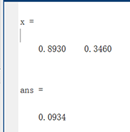Matlab代码：

A=[    0.8832    0.4020

0.7994    0.5721

0.7423    0.3525

0.8083    0.6609

1.1192    0.3386

1.1243    0.3069

1.1675    0.3462

1.1713    0.3360

0.9009    0.4236

1.1294    0.3259

0.8933    0.3767

0.1179    0.0353

1.1142    0.3195

0.8806    0.4084

0.7639    0.1711

0.1661    0.0556

1.0570    0.4109

0.7144    0.3081

0.8857    0.3881

1.0875    0.3538

1.1002    0.3272

0.7613    0.2244

1.0177    0.3525

];

a=sum(A(:,1))/23;

b=sum(A(:,2))/23;

plot(A(:,1),A(:,2),'x','LineWidth',2)%所有案发点坐标图

hold on

plot(a,b,'-ro',0.8930,0.3460,'*',0.88,0.42,'+')%画出模型

hold on                                        %居住点

x0=0.8930;y0 =0.346;

theta=0:pi/100:2*pi;

R=0.2334/2.5;

x=R*cos(theta)+x0;y=R*sin(theta)+y0;

plot(x,y,'-')

axis equal

hold on

x0=a;y0 =b;

theta=0:pi/100:2*pi;

R=0.2334/2.5;

x=R*cos(theta)+x0;y=R*sin(theta)+y0;

plot(x,y,'-')

axis equal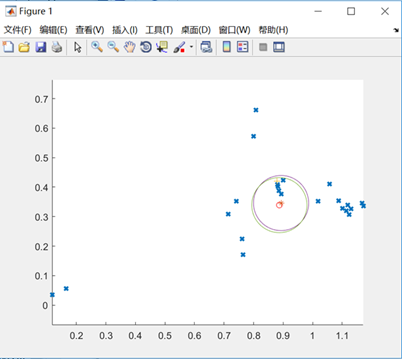5

4Rossmo模型求解：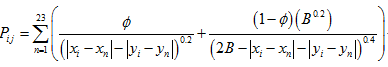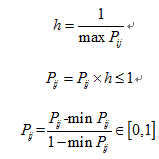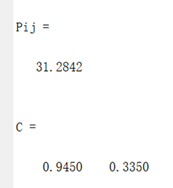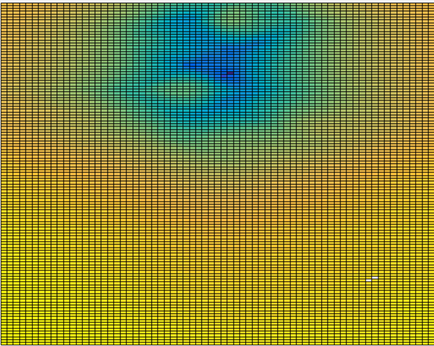6